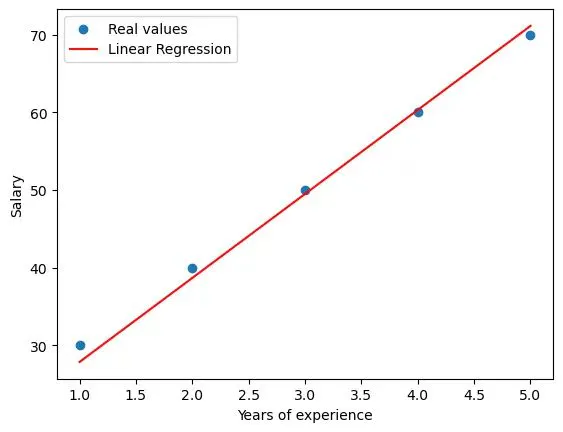How to Implement Gradient Descent with a Linear Regression Modelby@walterwhites

# How to Implement Gradient Descent with a Linear Regression ModelThis tutorial demonstrates how to create a simple linear regression model with gradient descent in Python. It explains key concepts like linear relationships, gradient descent, learning rate, and coefficients. The step-by-step guide includes code for essential functions and visualizing cost evolution with matplotlib, allowing you to predict salaries based on years of experience.### @walterwhites

Flo